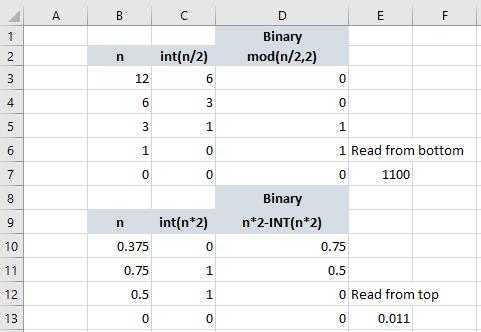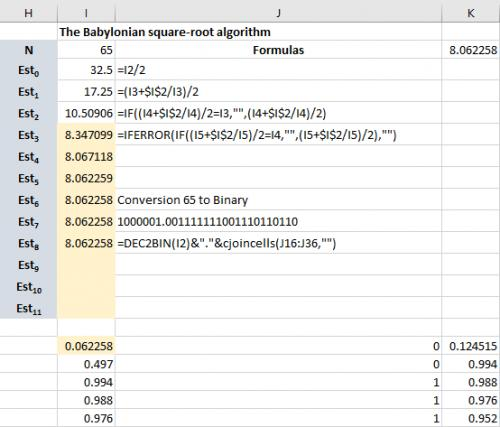Please Note: This article is written for users of the following Microsoft Excel versions: 2007, 2010, 2013, and 2016. If you are using an earlier version (Excel 2003 or earlier), this tip may not work for you. For a version of this tip written specifically for earlier versions of Excel, click here: Finding the Nth Root of a Number.

# Finding the Nth Root of a Numberby Allen Wyatt
(last updated November 9, 2020)

You may be wondering how to use Excel to derive different roots of a number. Finding a square root is easy: you just use the SQRT function. For instance, the following returns the square root of the value in cell B7:

```=SQRT(B7)
```

What about different roots, however? What if you want the fifth root of the value in B7, instead of the square root? Unless you are a math whiz (and I am not), the answer may not be that obvious. All you need to do is raise the value to the power of 1/n. For instance, if you want that fifth root of B7, then you would use the following formula:

```=B7^(1/5)
```

ExcelTips is your source for cost-effective Microsoft Excel training. This tip (12350) applies to Microsoft Excel 2007, 2010, 2013, and 2016. You can find a version of this tip for the older menu interface of Excel here: Finding the Nth Root of a Number.

##### Author Bio

Allen Wyatt

With more than 50 non-fiction books and numerous magazine articles to his credit, Allen Wyatt is an internationally recognized author. He is president of Sharon Parq Associates, a computer and publishing services company. ...

##### MORE FROM ALLEN

One of the easiest ways to quickly access a macro is to assign it to the Quick Access toolbar. Here's how you can make ...

Discover More

Automatic Lines for Dividing Lists

When preparing a report for others to use, it is not unusual to add a horizontal line between major sections of the ...

Discover More

Extracting INCLUDEPICTURE File Names

If you use the INCLUDEPICTURE field to add images to your document, you may love the macro in this tip. It allows you to ...

Discover MoreProfessional Development Guidance! Four world-class developers offer start-to-finish guidance for building powerful, robust, and secure applications with Excel. The authors show how to consistently make the right design decisions and make the most of Excel's powerful features. Check out Professional Excel Development today!

##### More ExcelTips (ribbon)

Phantom Counts

Two common worksheet functions used to count things are COUNT and COUNTA. Not understanding how these functions treat ...

Discover More

Using a Week Number as One Criterion in a Formula

The SUMIFS function can be quite powerful in conditionally summing information based on criteria you specify. This tip ...

Discover More

Excel allows you to easily convert values from decimal to other numbering systems, such as hexadecimal. This tip explains ...

Discover More
##### Subscribe

FREE SERVICE: Get tips like this every week in ExcelTips, a free productivity newsletter. Enter your address and click "Subscribe."

If you would like to add an image to your comment (not an avatar, but an image to help in making the point of your comment), include the characters [{fig}] in your comment text. You’ll be prompted to upload your image when you submit the comment. Maximum image size is 6Mpixels. Images larger than 600px wide or 1000px tall will be reduced. Up to three images may be included in a comment. All images are subject to review. Commenting privileges may be curtailed if inappropriate images are posted.

What is three less than 9?

2020-11-13 06:46:18

Peter Atherton

Ronald Finnerty

https://www.exploringbinary.com/binary-converter/
https://indepth.dev/the-simple-math-behind-decimal-binary-conversion-algorithms

Unfortunately, neither how to get the square root of a binary number. Here are the basics:

(see Figure 1 below)

You can convert a whole number to binary using the DEC2BIN function or by an algorithm shown in Fig1. It also shows how to convert a decimal to binary. Some decimals can not be converted exactly, so we stop when you get a repeated pattern.

For the decimal algorithm, you can use the TEXTJOIN function to get the decimal part from the algorithm.

To get a square root you can use the Babylonian algorithm {fig2), I just used the SQRT function in K2 of 65 to get the integer binary. And used a version of the TEXTJOIN for the decimal part.

(see Figure 2 below)

Of course, you don't need the Babylonian algorithm for the fraction just get it from the decimal SQRT function.Figure 1. Integer & Faction convertsion.Figure 2. Babylonian Fraction Algorithm

2020-11-12 05:47:03

JMJ

I'm afraid there is serious confusion here:

- n^(1/5) gives the fifth root of n
- n^-(5 ) gives the inverse of n^5

By example, if n = 10,
n^2 = 100
n^-2 = 0.01
n^(1/2) = 3.16227766 (this is the square root of 10)

So, using fractional exponents or negatives exponents is COMPLETELY DIFFERENT !

2017-09-25 10:20:04

Ronald Finnerty

this did not answer the finding nth root question. It merely shows how nth root calculation work.
If I have a number and need to find the nth root of base 2 of the number i am no further along with your solution.
i only know the answer is 2^1/n so how do i find n?

2017-06-20 09:22:53

Dennis Costello

Just to be pedantic, I'll point out that Maarten is almost correct. He said:
2^-1 for instance is 0.5 (2/2^1)
2^-3 is 0.125 (2/2^3)
When he meant
2^-1 for instance is 0.5 (1 / 2^1)
2^-3 is 0.125 (1 / 2^3)

2016-06-30 17:20:39

Maarten Daams

You can even use negative exponents.
2^-1 for instance is 0.5 (2/2^1)
2^-3 is 0.125 (2/2^3)
etc.
And so on.
Of course fractional negative exponents work also, but that is more complicated

2016-06-11 06:08:43

Polarisking

The POWER function also works here,

POWER(B7,1/5)

##### This Site

Got a version of Excel that uses the ribbon interface (Excel 2007 or later)? This site is for you! If you use an earlier version of Excel, visit our ExcelTips site focusing on the menu interface.